Showing Page:
1/11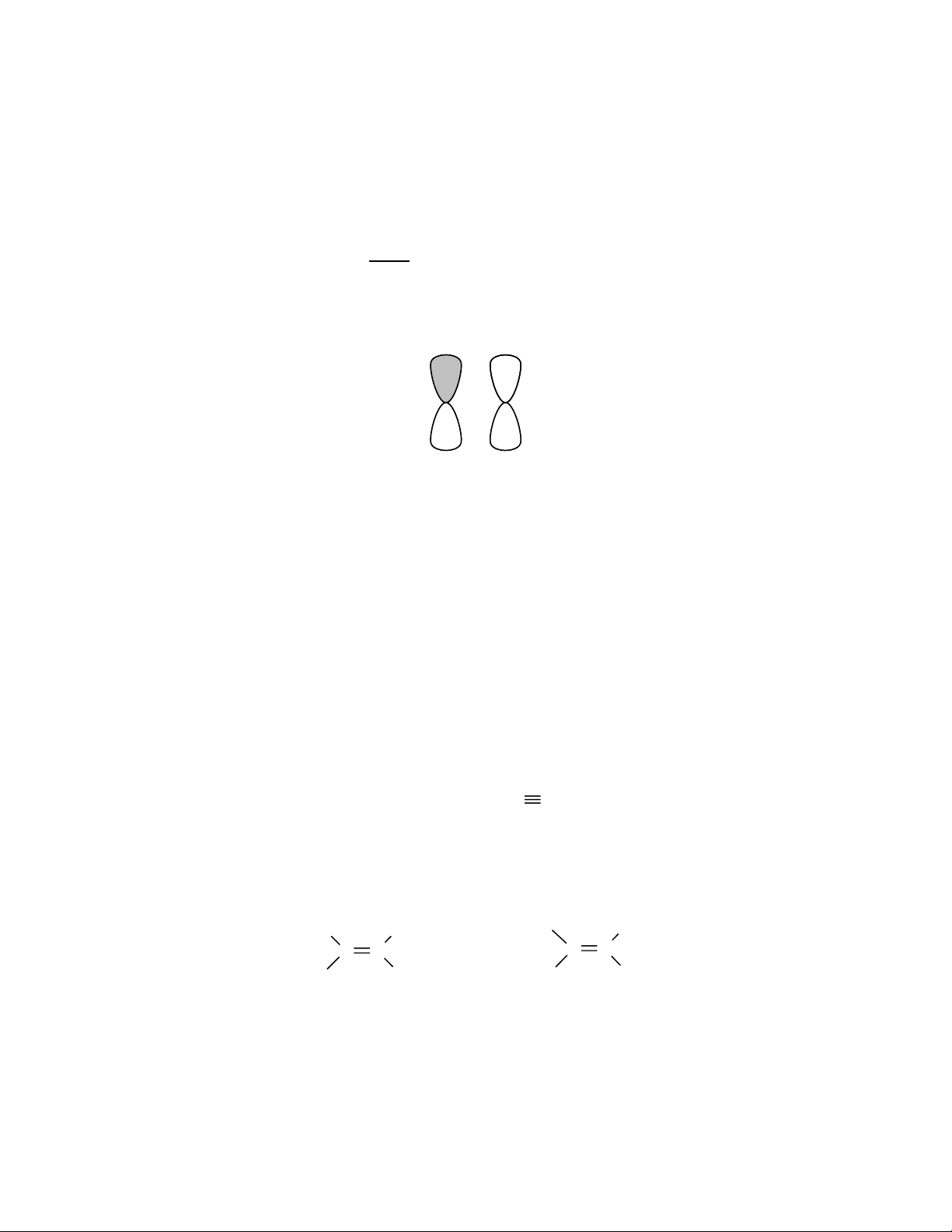Practice Questions for 32-235 Exam 1
Note these are for reviews only. Make sure that you can do all homework without
consulting the Solutions Manual and that you can complete them in a reasonable
amount of time (speed of essence).
Multiple Choices (Choose only ONE answer !)
1. What kind of molecular orbital results when the two atomic orbitals shown below
interact in the manner indicated?
(a) * (b) (c)* (d) (e) none of the above
2. Which of the following matches of names and molecules are correct ?
A. Alcohol I. HCOOH
B. Aldehyde II. (CH
3
)
3
COH
C. Ketone III. CH
3
OCH
3
D. Acid IV. CH
3
COCH
3
(a) C and III, D and IV (b) A and II, C and IV
(c) A and III, B and IV (d) B and II, D and I
(e) none of the above
3. The number of bonds in the molecule shown below is
CH
3
CH=CHCH
2
C CH
(a) 1 (b) 2 (c) 3 (d) 4 (e) 5
4. The relationship of the following two structures is
C C
H
3
C
CH
3
Br
Br
C C
H
3
C
CH
3
Br
Br
(a) structural isomers (b) geometric isomers (c) the same
(d) not isomeric (e) conformational isomers
Showing Page:
2/11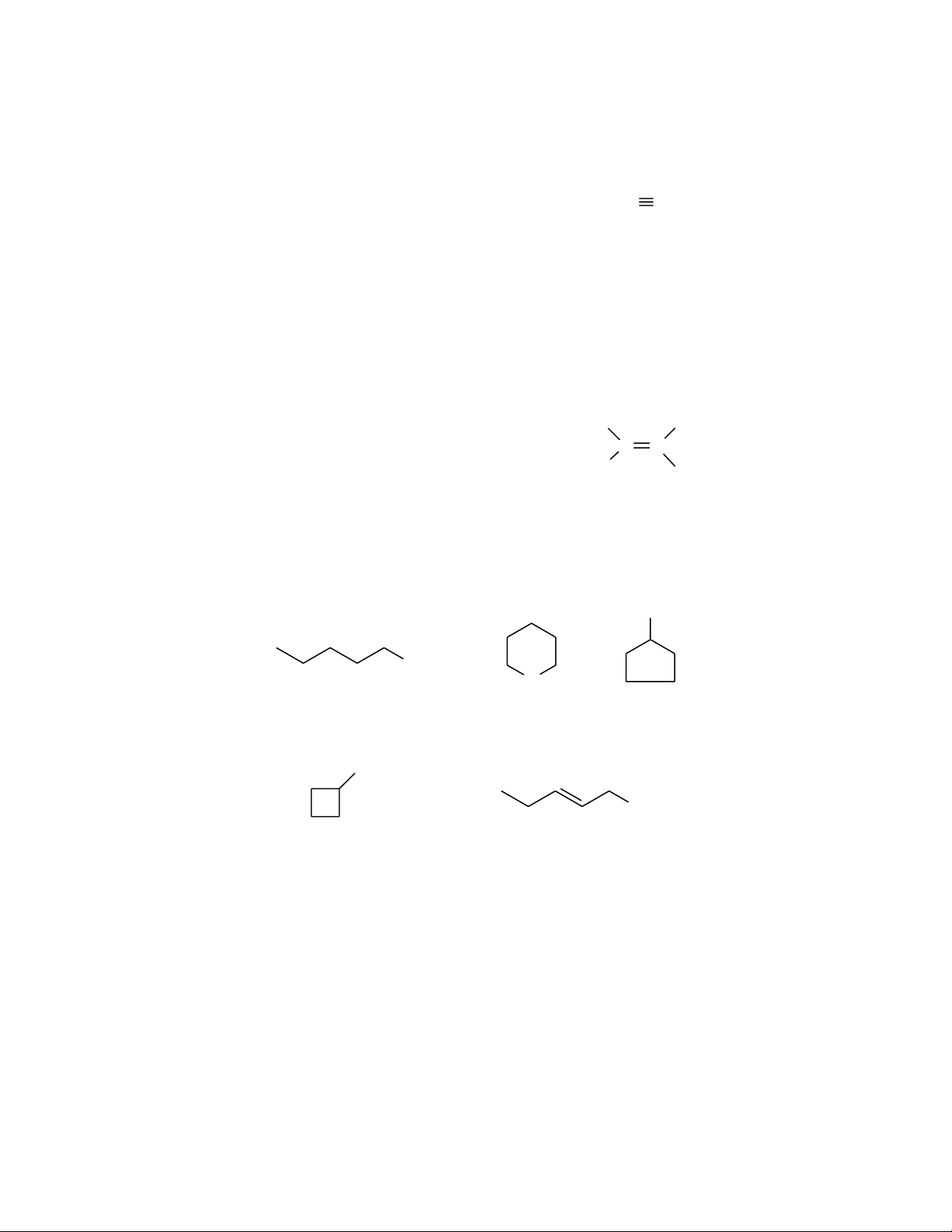Page 2 of 11
5. Which are the correct orbital hybridizations for the carbon atoms in the following
structures ?
CH
3
CH
3
CH
2
=CH
2
CH
3
+
HC N
H
2
O
sp
2
sp
2
sp
2
spsp
3
I
II
III
IV
V
(a) I, II, III (b) I, II, IV (c) II, III, V (d) III, IV, V
(e) I, III, V
6. Which of the following compounds is nonpolar (dipole moment = 0) ?
H
2
O NH
3
CH
3
Cl C C
Cl
Cl
H
H
(a)
(b) (c)
(d)
(e) none of the above
7. Which compound would have the lowest boiling point ?
O
OH
OH
CH
2
OH
OH
(a)
(b)
(c)
(d)
(e)
8. Consider the three isomeric alkanes n-hexane, 2,3-dimethylbutane, and 2-
methylpentane. Which of the following correctly lists these compounds in order
of increasing boiling point?
a) 2,3-dimethylbutane < 2-methylpentane < n-hexane
b) 2-methylpentane < n-hexane < 2,3-dimethylbutane
c) 2-methylpentane < 2,3-dimethylbutane < n-hexane
d) n-hexane < 2-methylpentane < 2,3-dimethylbutane
d) n-hexane < 2,3-dimethylbutane < 2-methylpentane
Showing Page:
3/11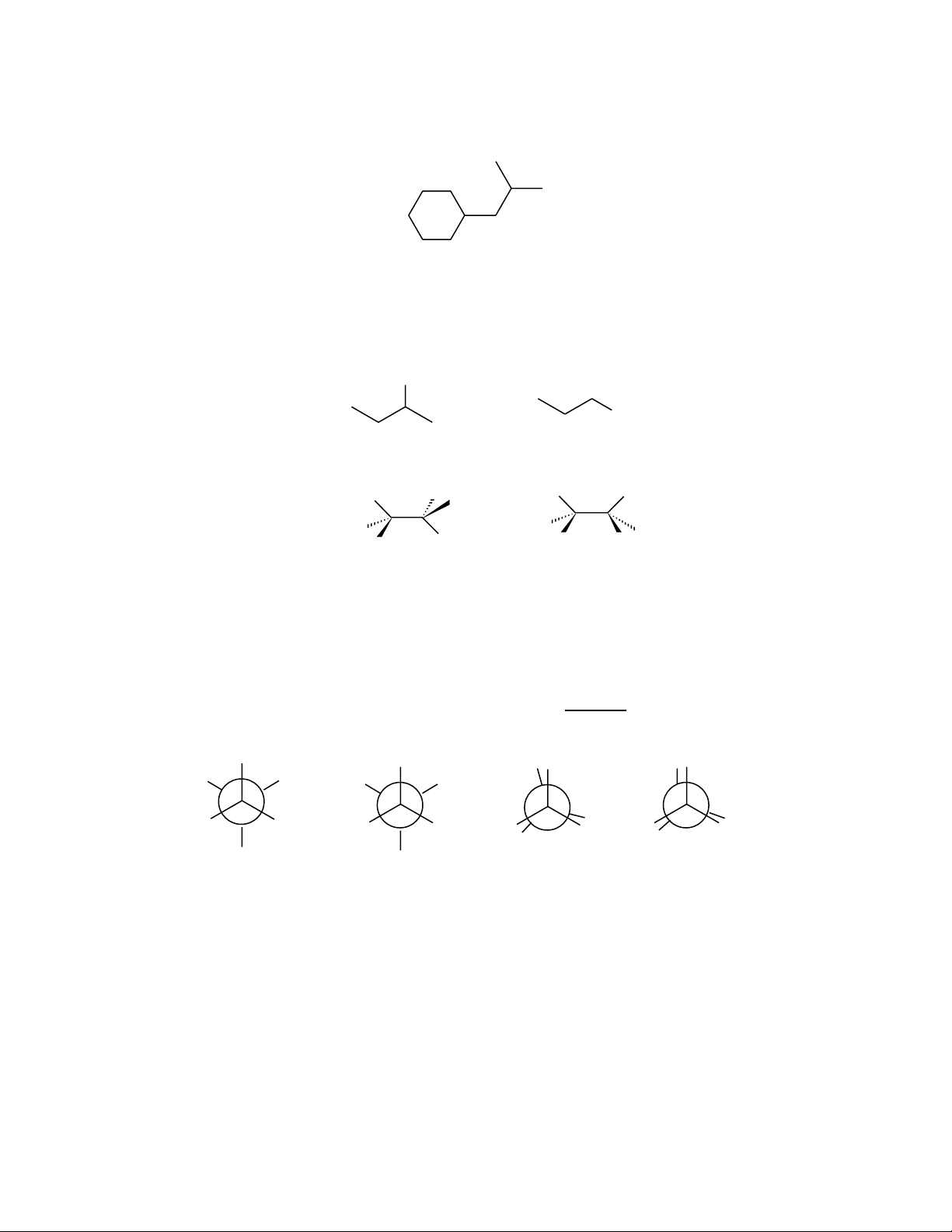Page 3 of 11
9. How many tertiary hydrogens are there in the following structure:
(a) 1 (b) 2 (c) 3 (d) 4 (e) 5
10. Which of the following pairs of formula represent structural isomers ?
F
F
F
F
H
F
H
H
F
H
F
H
H
F
CH
3
CH
2
CH
2
NH
2
(CH
3
)
3
N
and
and
and
(a)
(b)
(c)
d) More than one of these
e) None of these
11. Which of the following is a representation of the gauche conformer of butane.
CH
3
H H
H H
CH
3
CH
3
H H
H
3
C H
H
HH
CH
3
H
3
C
H
H
HH
3
C
H
H
CH
3
H
(a)
(b)
(c) (d)
(e) none of the above
12. Which of the above conformations is the least stable ?
(a) (b) (c) (d) (e)
Showing Page:
4/11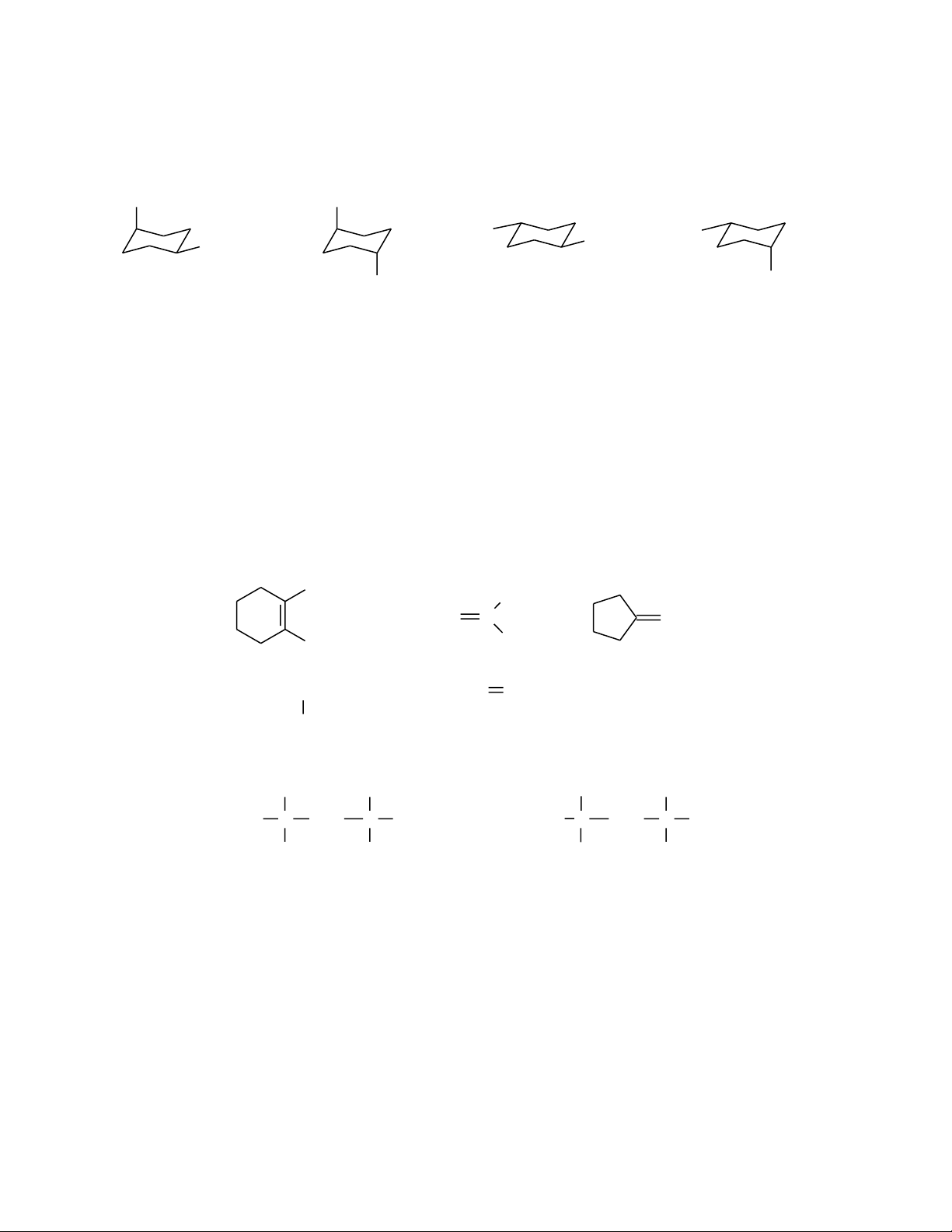Page 4 of 11
13. Which drawing represents the most stable conformation of cis-1-tert-butyl-4-
methylcyclohexane.
CH
3
C(CH
3
)
3
CH
3
C(CH
3
)
3
CH
3
C(CH
3
)
3
CH
3
C(CH
3
)
3
(a)
(b)
(c)
(d)
(e) none of the above
14. If stronger bonds are broken and weaker bonds are formed, then the reaction is
(a) is exothermic (b) is endothermic (c) has a positive
(d) has a negative (e) is spontaneous
15. Which of the following molecules can exist as geometric isomers?
16. The relationship between the following two structures is
C CH
2
C H
H
CH
3
H
H
CH
2
CH
3
C CH
2
C H
H
CH
3
H
H
CH
3
CH
2
(A) same (B) constitutional isomers (C) geometric isomers
(D) not isomers (E) structural isomers
Showing Page:
5/11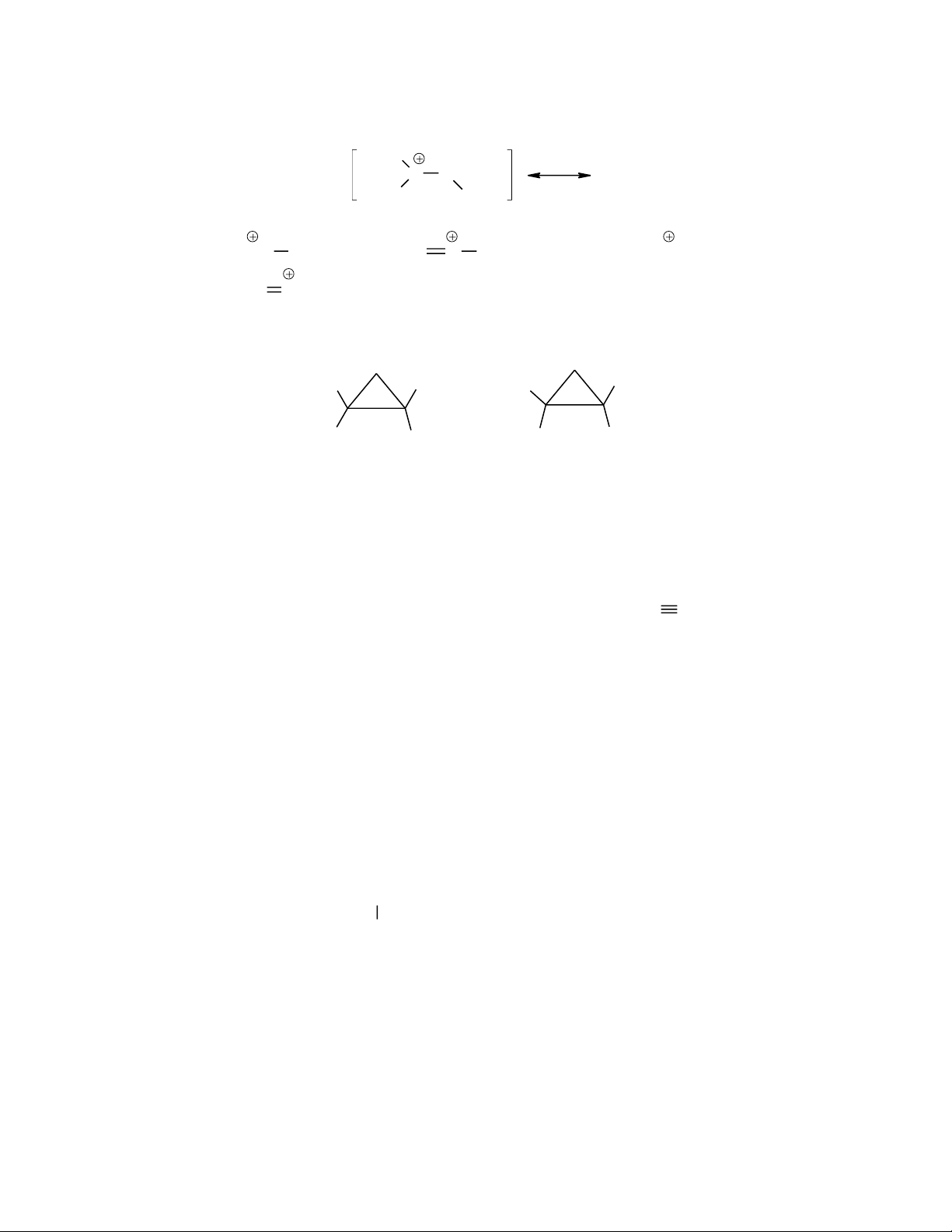Page 5 of 11
17. Which is an acceptable resonance structure for the following drawing?
C
H
H
O
CH
3
(E) None of the above
CH
3
CH OH
(A)
CH
2
O CH
3
(B)
CH
3
CH
2
O(C)
CH
3
CH OH(D)
18. The relationship of the following two structures is
H
3
C CH
3
H
H
H
3
C
CH
3
H
H
(A) structural isomers (B) conformational isomers (C) the same
(D) not isomeric (E) stereoisomers
19. Which is(are) the correct orbital hybridization(s) for the C and N atoms in the
following structures ?
CH
3
CH
3
CH
2
=CH
2
NH
3
HC CH
sp
sp
2
sp
3
I II III IV
sp
2
(A) I (B) II, III (C) III, IV (D) I, III (E) II
20. The b.p. of the following compounds is expected to decrease in this order
(highest b.p. first):
CH
3
CH
2
CH
2
CH
3
CH
3
CH
2
CH
2
CH
2
CH
3
CH
3
CH
2
CH
2
CH
2
OH
CH
3
CH
2
CHCH
3
CH
3
I
II
III
IV
(A) II > III > IV > I (B) III > I > II > IV (C) III > II > IV > I
(D) IV > II > III > I (E) I > II > III > IV
Showing Page:
6/11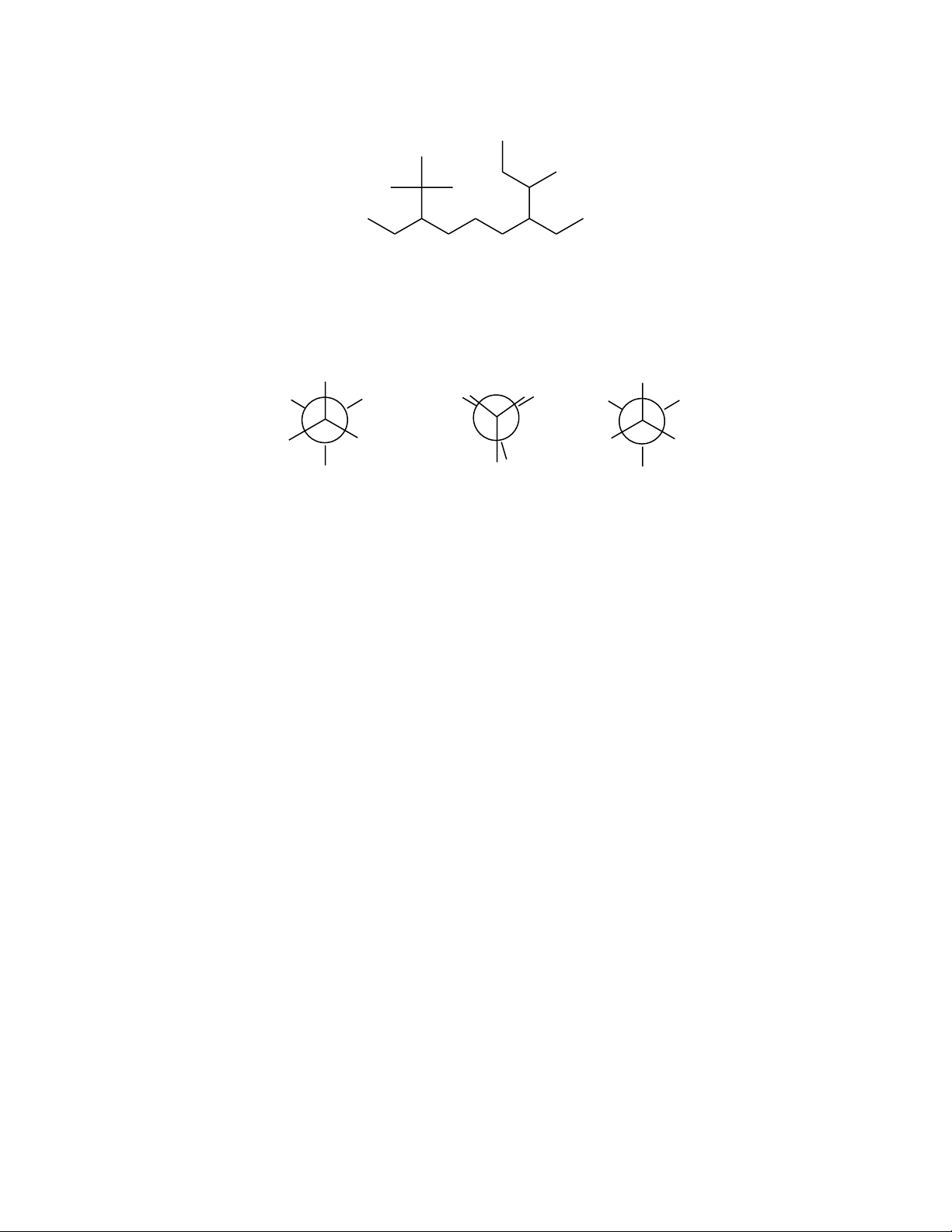Page 6 of 11
21. An acceptable name for the following structure is
(A) 8-t-Butyl-4-ethyl-3-methyldecane (B) 3-sec-Butyl-7-tert-butylnonane
(C) 3,7-Diethyl-2,2,8-trimethyldecane (D) 4,8-Diethyl-3,9,9-trimethyldecane
(E) none of the above
22. Which statement is true regarding the following conformations for butane?
H
CH
3
H
H H
CH
3
CH
3
H H
H H
CH
3
H
H
3
C
H
H H
CH
3
I II III
(A) I is less stable than II because of steric strain
(B) III is less stable than I due to its torsional strain
(C) III is called gauche conformation
(D) The steric strain in II is greater than in I
(E) III has more energy than II
Showing Page:
7/11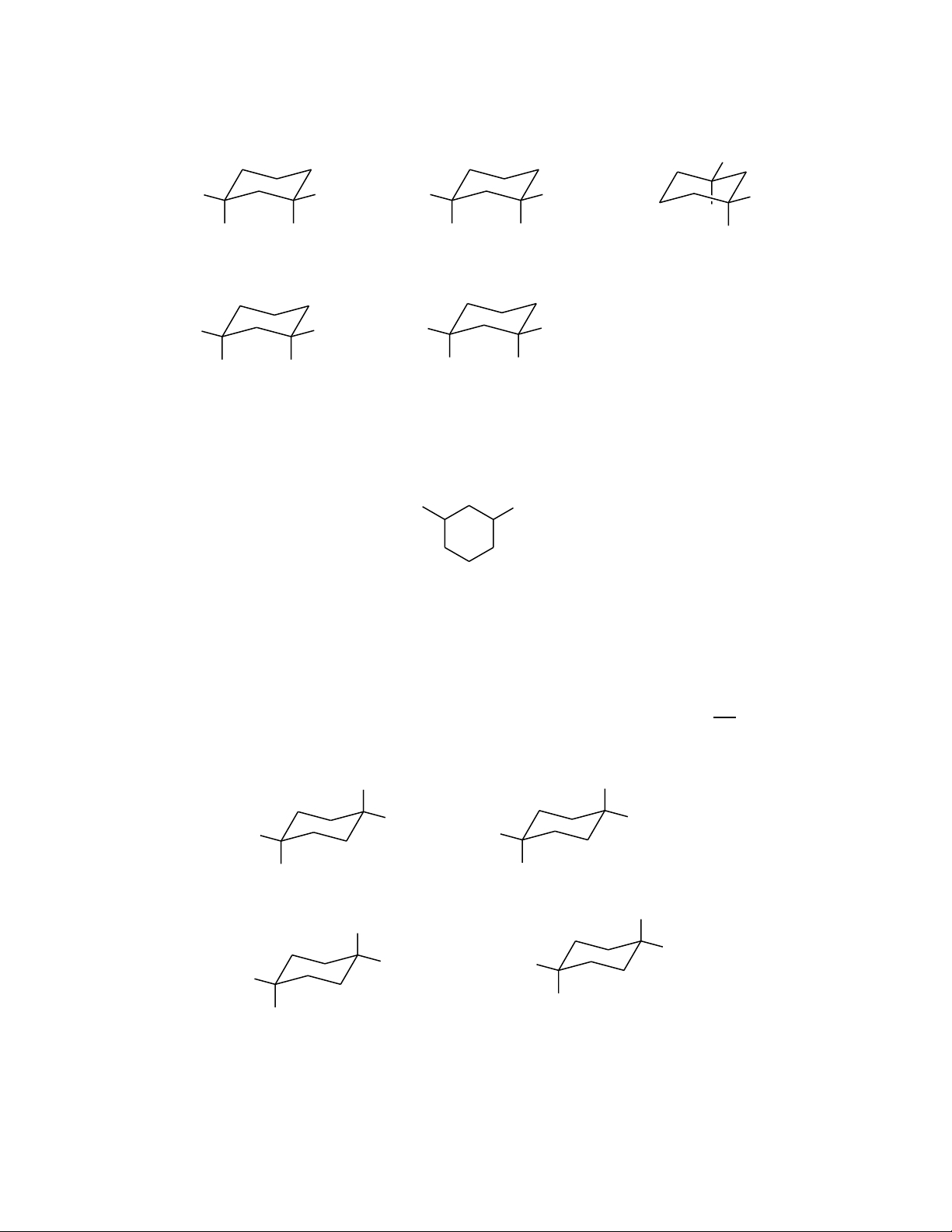Page 7 of 11
23. Which of the following molecules is the least stable ?
H
CH
3
H
CH
3
H
CH
3
CH
3
H
H
3
C
H H
CH
3
H
3
C
H CH
3
H
H
CH
3
H
CH
3
(A)
(B)
(C)
(D)
(E)
24. Which name(s) is(are) acceptable for the following compound?
Cl CH
3
(A) 5-chloro-2-methylcyclohexane
(B) 4-chloro-2-methylcyclohexane
(C) 1-chloro-3-methylcyclohexane
(D) 2-chloro-5-methylcyclohexane
(E) More than one of these
25. Which of the following represents the most stable conformation of cis-1-
isopropyl-4-methylcyclohexane?
(D)
(C)
(B)
(A)
CH
3
CH(CH
3
)
2
H
H
H
H
H
3
C
CH(CH
3
)
2
CH
3
H
H
CH(CH
3
)
2
H
CH(CH
3
)
2
H
3
C
H
(E) None of these fits the name given
Showing Page:
8/11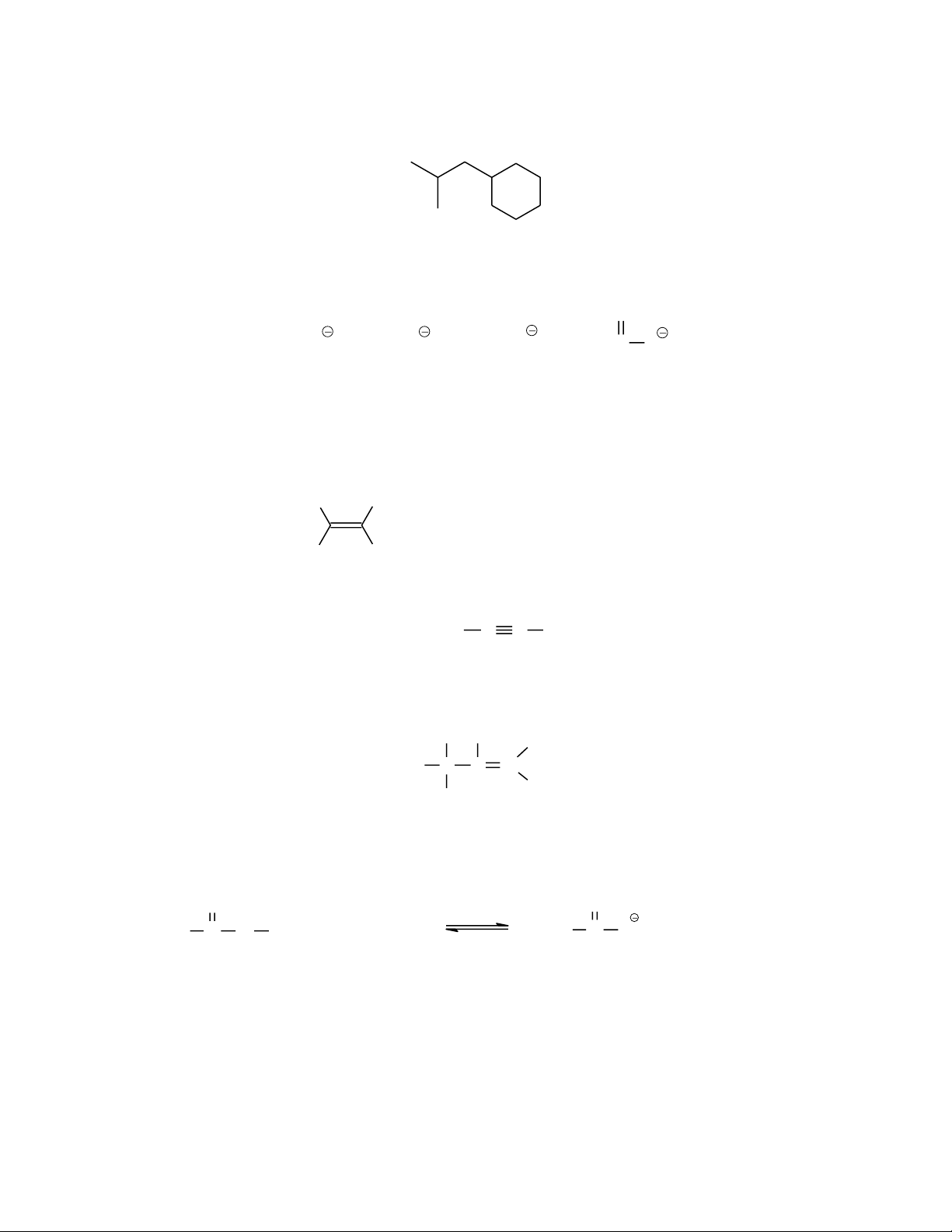Page 8 of 11
26. How many tertiary hydrogen(s) are(is) there in the following structure?
(A) 1 (B) 2 (C) 3 (D) 6 (E) 10
27. The strength of the following bases decreases in the order of (strongest first):
CH
3
O CH
3
NH
2
CH
3
C O
O
I II III IV
(A) I > IV > III > II (B) III > IV > I > II (C) II > I > III > IV
(D) IV > I > II > III (E) II > III > I > IV
28. Which molecule is expected to have the largest molecular dipole moment ?
H
Cl
Cl
H
BH
3
CH
3
Cl
CCl
4
C C FF
(A)
(B)
(C)
(D)
(E)
29. The formal charge on nitrogen in the Lewis structure below is
C C N
H
H
HH
H
H
(A) +2 (B) +1 (C) 0 (D) -1 (E) -2
30. Considering the following acid-base reaction, which statement is NOT true?
pK
a
=9.2
pK
a
= 4.7
+
CH
3
C
O
O H
+
CH
3
C
O
O
I II
III
IV
NH
3
NH
4
+
(A) I is more acidic than IV
(B) II is more basic than III
(C) The equilibrium favors the right
(D) The two C-O bonds in III are of different length.
(E) more than one of the above
Showing Page:
9/11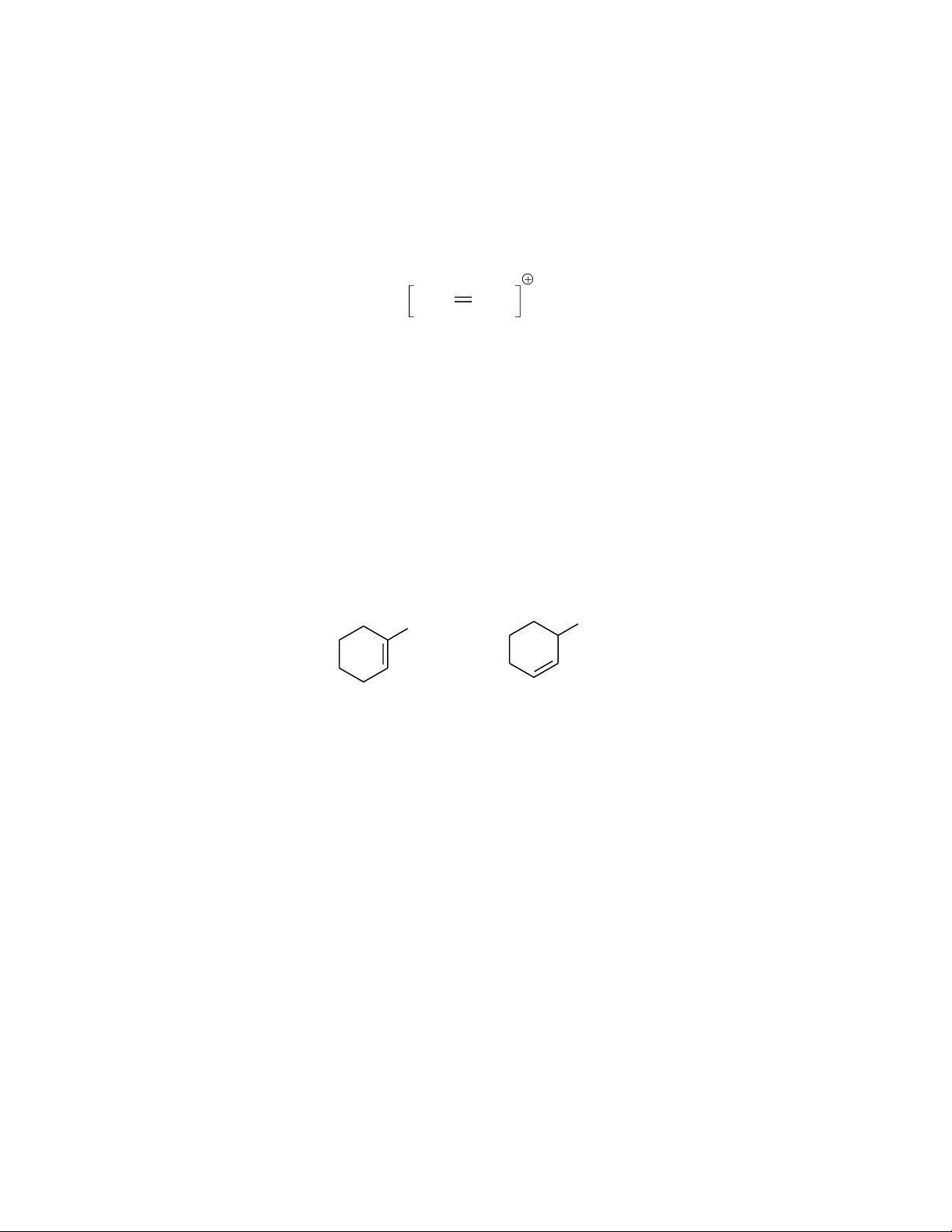Page 9 of 11
31. When the 1s orbitals of two hydrogen atoms combine to form a hydrogen
molecule, how many molecular orbitals are formed ?
(A) one (B) two (C) three (D) four (E) five
32. The nitrogen atom in the molecule below is sp
2
hybridized. What is the
geometry of the atoms connected to nitrogen ?
CH
2
NH
2
(A) square (B) tetrahedral (C) trigonal planar
(D) linear (E) pyramidal
33. Which of the following is the best description for the C=N bond above?
(A) It results from sp
2
(C)-sp
2
(N) overlap
(B) It results from sp
3
(C)-sp
2
(N) overlap
(C) The two bonds are of same property
(D) One bond is from sp
2
(C)-sp
2
(N) and the other from 2 p orbitals
(E) None of the above
34. The relationship of the following two molecules is best described by
CH
3
CH
3
(A) structural isomers (B) geometric isomers
(C) not isomers (D) homologous
(E) conformational isomers
35. Which of the following bonds can act to form hydrogen bonding
(A) O-H (B) N-H (C) C-H (D) C-C
(E) more than one the above
36. The boiling point of straight chain alkanes increases as the number of carbons
increases. This is because
(A) the larger the molecule, the greater the dipole moment
(B) Larger molecules usually can form hydrogen bonding better
(C) Larger molecules can pack tightly together
(D) Larger molecules have greater surface therefore stronger London Forces
among them.
(E) None of the above
Showing Page:
10/11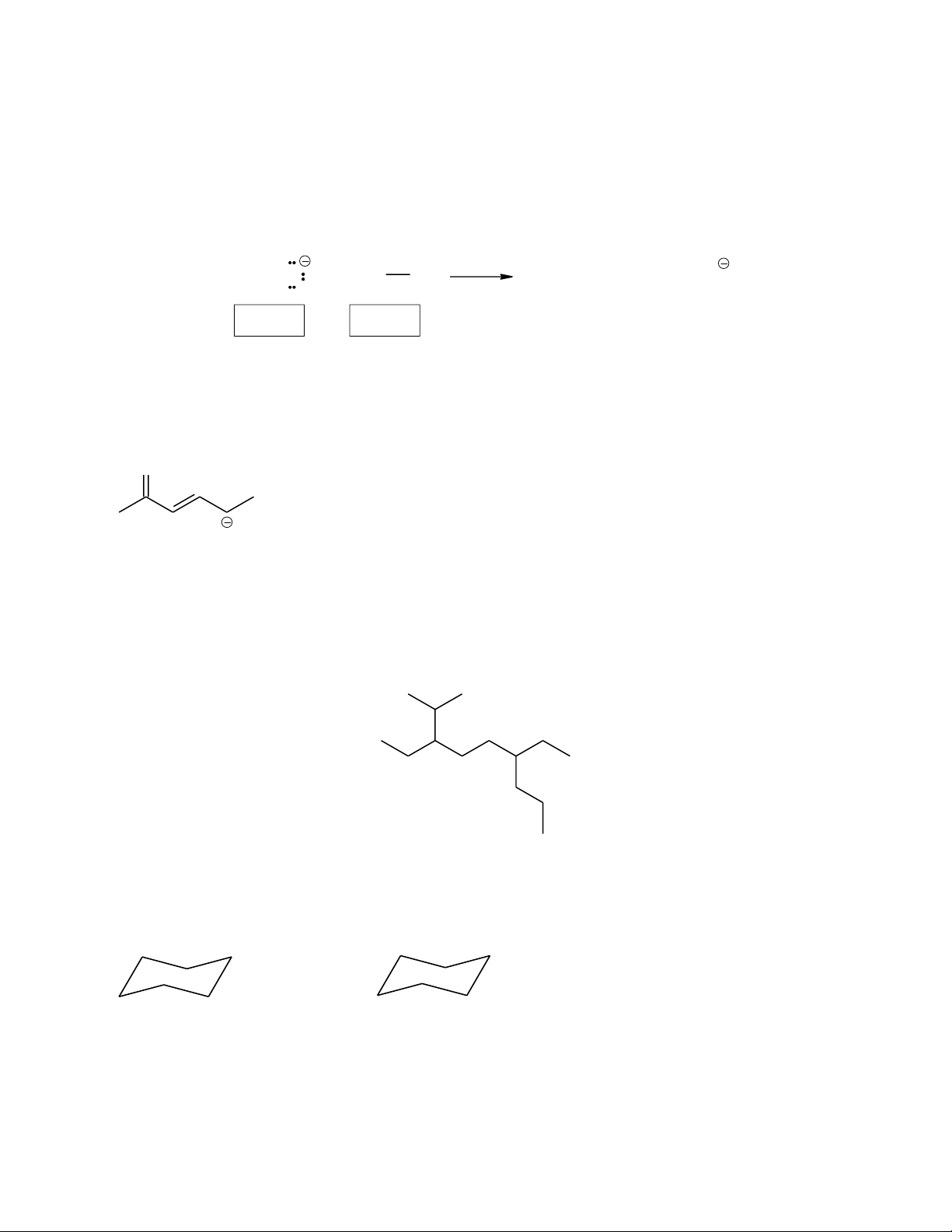Page 10 of 11
Essay Questions
1. Use the curved arrow formalism(electron pushing) to indicate the movement of
electron pairs in the following reaction. Indicate in the boxes which reactants are
nucleophile (Nu) and/or electrophile (E).
CH
3
CH
2
O + CH
3
I
CH
3
CH
2
OCH
3
+ I
2. Draw the important resonance contributing forms for the structure shown below.
(Use electron arrow pushing for help)
O
3. Give an acceptable IUPAC name for the following compound:
4. (A) Write the two chair conformations for cis-1-tert-butyl-2-ethylcyclohexane (the following
A
B
(B) In the above conformations, label the positions occupied by tert-butyl and ethyl groups by
using "ax" for axial and "eq" for equatorial positions.
Showing Page:
11/11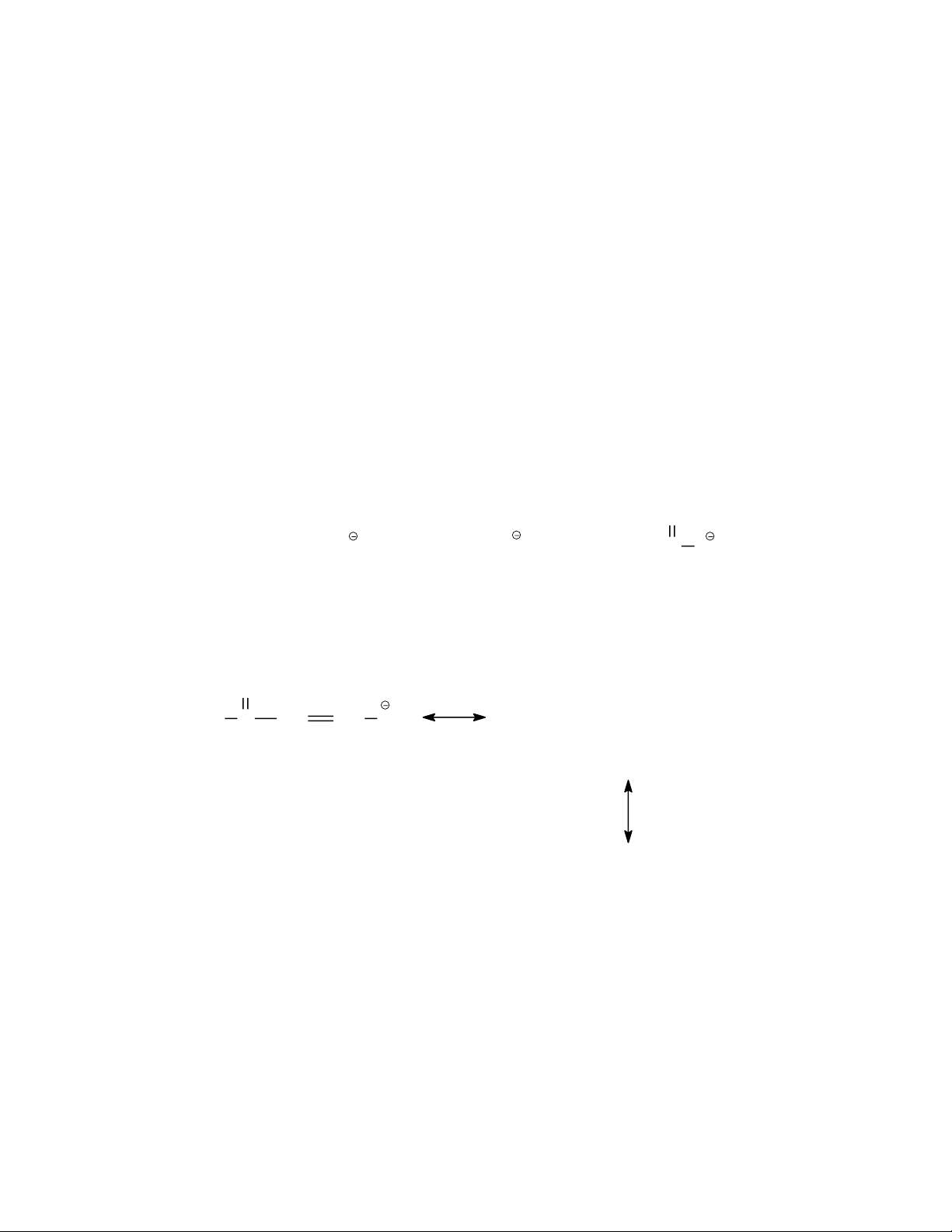Page 11 of 11
(C) Determine which conformation would have a higher energy and briefly explain the reason.
5. The three compounds below have identical or nearly identical molecular mass. Rank them in
the increasing order (lowest first) of their boiling points, and briefly explain your reasoning.
6. Draw the Lewis structures for three compounds with a formula of C
3
H
8
O. What is the
relationship among these three compounds?
7. Redraw each molecule below to show lone pair electrons, then arrange them in the order of
increasing basicity (lowest first, use <). Explain your reasoning for the order.
8. Draw the important resonance structures for the following molecule. (hint: try electron arrow
pushing)
CH
3
CH
2
O CH
3
CH
2
NH
CH
3
C
O
O
A B C
H C
O
CH CH CH
2
CH
3
CH
2
CH
2
CH
2
OH CH
3
CH
2
OCH
2
CH
3
HOCH
2
CH
2
OH
A B C

### Unformatted Attachment Preview

Practice Questions for 32-235 Exam 1 Note these are for reviews only. Make sure that you can do all homework without consulting the Solutions Manual and that you can complete them in a reasonable amount of time (speed of essence). Multiple Choices (Choose only ONE answer !) 1. What kind of molecular orbital results when the two atomic orbitals shown below interact in the manner indicated? (a) * 2. (b)  (c) * I. HCOOH II. (CH3)3COH III. CH3OCH3 IV. CH3COCH3 (a) C and III, D and IV (c) A and III, B and IV (e) none of the above (b) A and II, C and IV (d) B and II, D and I The number of  bonds in the molecule shown below is CH3CH=CHCH2C CH (a) 1 4. (e) none of the above Which of the following matches of names and molecules are correct ? A. Alcohol B. Aldehyde C. Ketone D. Acid 3. (d)  (b) 2 (c) 3 (d) 4 (e) 5 The relationship of the following two structures is H3C Br Br C C C C Br (a) structural isomers (d) not isomeric Br CH3 H3C CH3 (b) geometric isomers (c) the same (e) conformational isomers 5. Which are the correct orbital hybridizations for the carbon atoms in the following structures ? CH2=CH2 sp2 CH3 sp2 HC N sp2 H2O sp I II III IV V (a) I, II, III (e) I, III, V 6. + CH3CH3 sp3 (b) I, II, IV (c) II, III, V (d) III, IV, V Which of the following compounds is nonpolar (dipole moment = 0) ? Cl Cl H2O NH3 CH3Cl C C H (b) (a) 7. (c) H (d) (e) none of the above Which compound would have the lowest boiling point ? OH OH (a) O (b) (c) CH2OH OH (d) 8. (e) Consider the three isomeric alkanes n-hexane, 2,3-dimethylbutane, and 2methylpentane. Which of the following correctly lists these compounds in order of increasing boiling point? a) b) c) d) d) 2,3-dimethylbutane < 2-methylpentane < n-hexane 2-methylpentane < n-hexane < 2,3-dimethylbutane 2-methylpentane < 2,3-dimethylbutane < n-hexane n-hexane < 2-methylpentane < 2,3-dimethylbutane n-hexane < 2,3-dimethylbutane < 2-methylpentane Page 2 of 11 9. How many tertiary hydrogens are there in the following structure: (a) 1 10. (b) 2 (c) 3 (d) 4 (e) 5 Which of the following pairs of formula represent structural isomers ? F (a) and (c) d) e) 11. F F HH F (b) F and F H CH3CH2CH2NH2 and F F HH H (CH3)3N More than one of these None of these Which of the following is a representation of the gauche conformer of butane. CH3 CH3 H H3C H H H H H CH3 H (a) (b) HH H3C CH3 H H H H H (c) HCH3 H3C H (d) (e) none of the above 12. Which of the above conformations is the least stable ? (a) (b) (c) (d) (e) Page 3 of 11 13. Which drawing represents the most stable conformation of cis-1-tert-butyl-4methylcyclohexane. CH3 CH3 CH3 C(CH3)3 CH3 C(CH3)3 C(CH3)3 C(CH3)3 (d) (c) (b) (a) (e) none of the above 14. If stronger bonds are broken and weaker bonds are formed, then the reaction is (a) is exothermic (d) has a negative S° 15. (c) has a positive S° (b) is endothermic (e) is spontaneous Which of the following molecules can exist as geometric isomers? CH3 H (B) CH2 (A) CHCH2CH3 Cl CH3 (D) CH3CH2CHCH3 (C) C (E) CH3CH CHCH3 Cl 16. The relationship between the following two structures is H H H H C CH2 CH2CH3 C H CH3 CH3CH2 C H CH3 CH2 C H H (A) same (B) constitutional isomers (C) geometric isomers (D) not isomers (E) structural isomers Page 4 of 11 17. 18. Which is an acceptable resonance structure for the following drawing? H C O H CH3 (A) CH3CH OH (B) CH2 (D) CH3CH OH (E) None of the above CH3 H H (A) structural isomers (D) not isomeric CH3 H H H3C (B) conformational isomers (E) stereoisomers (C) the same Which is(are) the correct orbital hybridization(s) for the C and N atoms in the following structures ? (A) I 20. (C) CH3CH2O The relationship of the following two structures is H3C 19. O CH3 CH3CH3 CH2=CH2 NH3 HC CH sp3 sp sp2 sp2 I II III IV (B) II, III (C) III, IV (D) I, III (E) II The b.p. of the following compounds is expected to decrease in this order (highest b.p. first): CH3CH2CH2CH3 I CH3CH2CH2CH2CH3 CH3CH2CHCH3 CH3CH2CH2CH2OH II CH3 IV III (A) II > III > IV > I (D) IV > II > III > I (B) III > I > II > IV (E) I > II > III > IV (C) III > II > IV > I Page 5 of 11 21. An acceptable name for the following structure is (A) 8-t-Butyl-4-ethyl-3-methyldecane (C) 3,7-Diethyl-2,2,8-trimethyldecane (E) none of the above 22. (B) 3-sec-Butyl-7-tert-butylnonane (D) 4,8-Diethyl-3,9,9-trimethyldecane Which statement is true regarding the following conformations for butane? H H H CH3 H CH3 (A) (B) (C) (D) (E) HH HH H3CCH3 I II I is less stable than II because of steric strain III is less stable than I due to its torsional strain III is called gauche conformation The steric strain in II is greater than in I III has more energy than II CH3 H H H H CH3 III Page 6 of 11 23. Which of the following molecules is the least stable ? CH3 H H H3C H H H CH3 CH3 H H CH3 H H H (E) Which name(s) is(are) acceptable for the following compound? Cl (A) (B) (C) (D) (E) 25. (C) H3C CH3 (D) 24. CH3 (B) (A) CH3 CH3 CH3 5-chloro-2-methylcyclohexane 4-chloro-2-methylcyclohexane 1-chloro-3-methylcyclohexane 2-chloro-5-methylcyclohexane More than one of these Which of the following represents the most stable conformation of cis-1isopropyl-4-methylcyclohexane? H CH(CH3)2 H H CH(CH3)2 H3C H CH3 (B) (A) H CH(CH3)2 H CH(CH3)2 H H3C H CH3 (C) (D) (E) None of these fits the name given Page 7 of 11 26. How many tertiary hydrogen(s) are(is) there in the following structure? (A) 1 27. (B) 2 (C) 3 (D) 6 (E) 10 The strength of the following bases decreases in the order of (strongest first): O CH3O CH3 I (A) I > IV > III > II (D) IV > I > II > III 28. NH2 II III (B) III > IV > I > II (E) II > III > I > IV IV (C) II > I > III > IV Which molecule is expected to have the largest molecular dipole moment ? H Cl Cl H (A) CCl4 F (D) 29. CH3C O BH3 CH3Cl (B) (C) C C F (E) The formal charge on nitrogen in the Lewis structure below is H H H H C C N H (A) +2 30. (B) +1 (C) 0 H (D) -1 (E) -2 Considering the following acid-base reaction, which statement is NOT true? CH3 O C O H + NH3 CH3 O C III I II pKa = 4.7 (A) I is more acidic than IV (B) II is more basic than III (C) The equilibrium favors the right (D) The two C-O bonds in III are of different length. (E) more than one of the above O + + NH4 IV pKa=9.2 Page 8 of 11 31. When the 1s orbitals of two hydrogen atoms combine to form a hydrogen molecule, how many molecular orbitals are formed ? (A) one 32. (B) two (C) three (E) five The nitrogen atom in the molecule below is sp2 hybridized. What is the geometry of the atoms connected to nitrogen ? CH2 (A) square (D) linear 33. (D) four (B) tetrahedral (E) pyramidal NH2 (C) trigonal planar Which of the following is the best description for the C=N bond above? (A) It results from sp2(C)-sp2(N) overlap (B) It results from sp3(C)-sp2(N) overlap (C) The two bonds are of same property (D) One bond is from sp2(C)-sp2(N) and the other from 2 p orbitals (E) None of the above 34. The relationship of the following two molecules is best described by CH3 CH3 (A) structural isomers (C) not isomers (E) conformational isomers 35. Which of the following bonds can act to form hydrogen bonding (A) O-H (B) N-H (E) more than one the above 36. (B) geometric isomers (D) homologous (C) C-H (D) C-C The boiling point of straight chain alkanes increases as the number of carbons increases. This is because (A) the larger the molecule, the greater the dipole moment (B) Larger molecules usually can form hydrogen bonding better (C) Larger molecules can pack tightly together (D) Larger molecules have greater surface therefore stronger London Forces among them. (E) None of the above Page 9 of 11 Essay Questions 1. Use the curved arrow formalism(electron pushing) to indicate the movement of electron pairs in the following reaction. Indicate in the boxes which reactants are nucleophile (Nu) and/or electrophile (E). CH3CH2O 2. + CH3 I CH3CH2OCH3 + I Draw the important resonance contributing forms for the structure shown below. (Use electron arrow pushing for help) O 3. Give an acceptable IUPAC name for the following compound: 4. (A) Write the two chair conformations for cis-1-tert-butyl-2-ethylcyclohexane (the following two templates are drawn to help you) A B (B) In the above conformations, label the positions occupied by tert-butyl and ethyl groups by using "ax" for axial and "eq" for equatorial positions. Page 10 of 11 (C) Determine which conformation would have a higher energy and briefly explain the reason. 5. The three compounds below have identical or nearly identical molecular mass. Rank them in the increasing order (lowest first) of their boiling points, and briefly explain your reasoning. CH3CH2CH2CH2OH CH3CH2OCH2CH3 HOCH2CH2OH A B C 6. Draw the Lewis structures for three compounds with a formula of C3H8O. What is the relationship among these three compounds? 7. Redraw each molecule below to show lone pair electrons, then arrange them in the order of increasing basicity (lowest first, use <). Explain your reasoning for the order. O CH3CH2O A CH3CH2NH B CH3C O C 8. Draw the important resonance structures for the following molecule. (hint: try electron arrow pushing) O H C CH CH CH2 Page 11 of 11 Name: Description: ...User generated content is uploaded by users for the purposes of learning and should be used following Studypool's honor code & terms of service.Studypool4.7Trustpilot4.5Sitejabber4.4